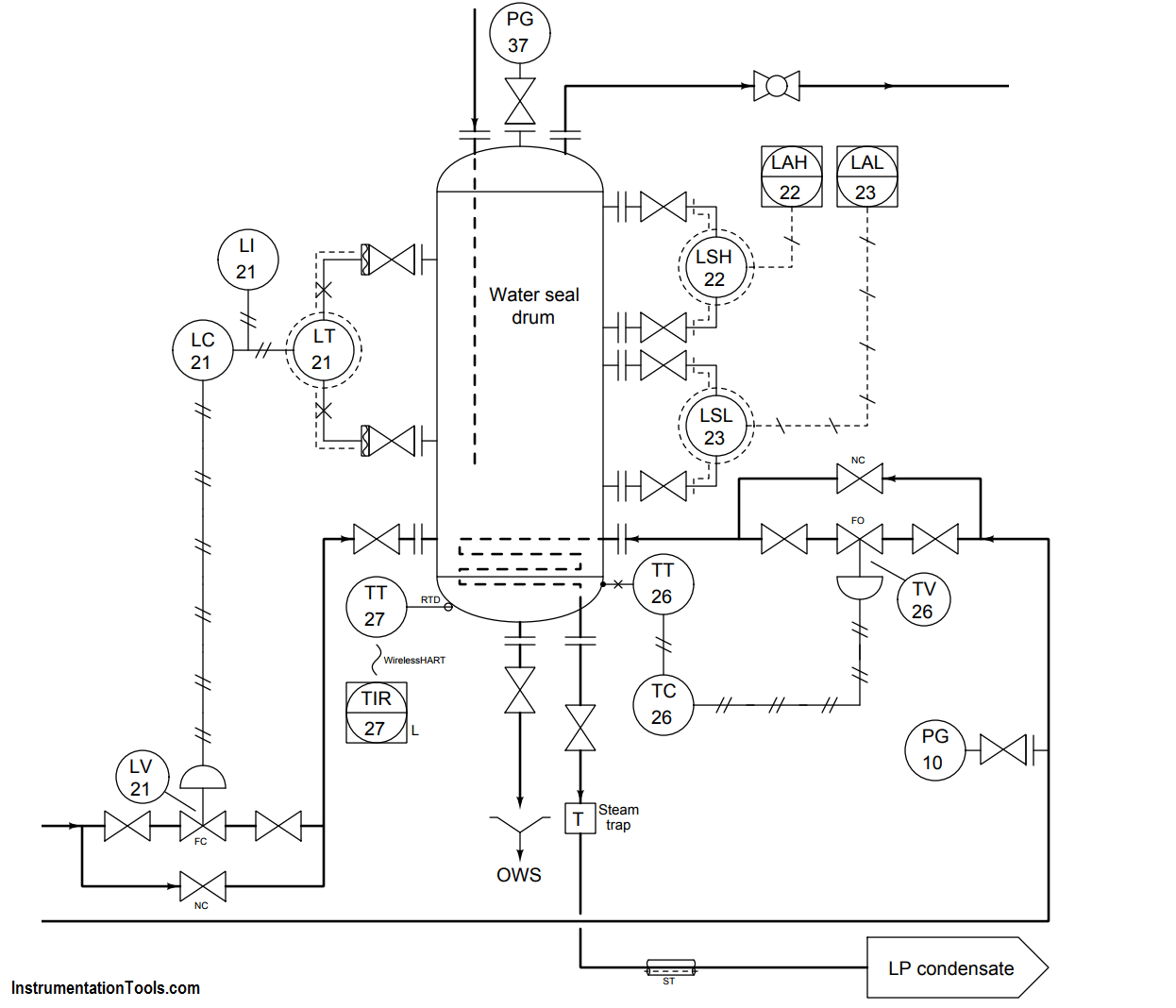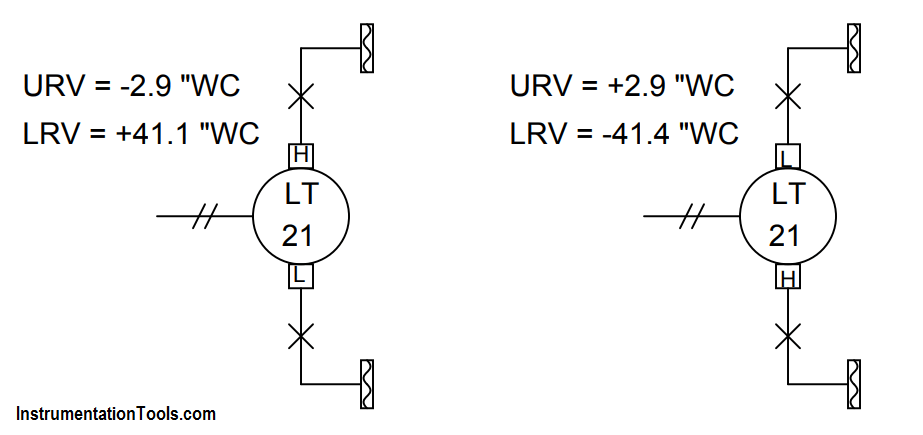# Determine LRV and URV settings for the Level Transmitter

#### Question :

Determine the LRV and URV settings for the water seal drum level transmitter (LT-21), assuming the LRV point is at the lower nozzle and the URV point is at the upper nozzle (the two nozzles being 3 feet 8 inches apart from each other), and that the remote seal fill fluid has a specific gravity of 0.934:The elevation for this transmitter (i.e. the total differential pressure applied by the height of fill fluid on both sides) is equal to the total height difference between the remote seal diaphragms multiplied by the specific gravity of the fill fluid:

Pelevation = (44 in)(0.934) = 41.1 ”WC

In the LRV condition, this is the only pressure seen by the transmitter. Therefore, 41.1 ”WC is the appropriate LRV setting for this transmitter. If we assume that the “H” port of this DP transmitter connects to the lower nozzle, the LRV will be -44.1 ”WC. If we assume the “H” port connects to the upper nozzle, the LRV will be +41.4 ”WC.

In the URV condition, we have the exact same amount of elevation (the fill fluid inside the capillary tubes) but on the lower nozzle we have the hydrostatic pressure of 44 vertical inches of water (i.e. the water inside the seal drum). Thus, in the URV condition the transmitter sees a differential pressure of:

Pdifferential = 44” WC − 41.1 ”WC = 2.9 ”WC

If we assume the “H” port of this DP transmitter connects to the lower nozzle, the URV will be +2.9 ”WC.

If we assume the “H” port of this DP transmitter connects to the upper nozzle, the URV will be -2.9 ”WC.1.